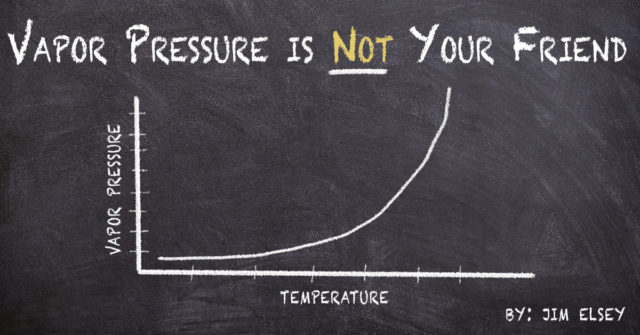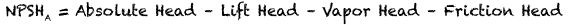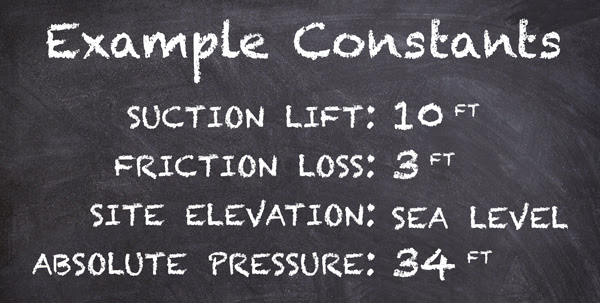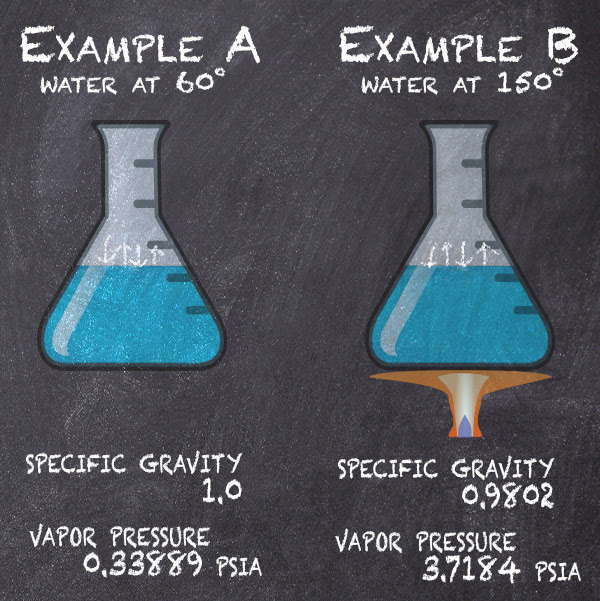1-800-489-8677 solutions@raross.com
Select Page#### Be very careful on self-primer lift applications because the liquid temperature directly affects its vapor pressure and that…

changes the Net Positive Suction Head Available (NPSHA).Example: Self-Primer – two temperatures… two outcomes:
For the example we will use two versions of the otherwise same application. The applications are identical in both versions except the temperature of the fluid is higher in the second version. The higher temperature signifies the vapor pressure has increased.*Note: 14.7 PSI absolute atmospheric pressure x 2.31 divided by the Specific Gravity of 1 = 33.96 feet ≈ 34).
Any increase in elevation will reduce your absolute pressure (head) and consequently the NPSHA.

In Example A the fluid is water at 68ºF.
In Example B the fluid is water at 150ºF
As you look up the vapor pressure of water for each temperature note it is normally expressed in PSIA (pounds per square inch absolute) and so you need to convert that value to feet (head) then you will have the component value in the proper units needed to do the NPSHA calculation. Remember that to convert PSI (or PSIA) to head, you must multiply by 2.31 and divide by the specific gravity.You can find these vapor pressure and specific gravity values in several places;
I use the Cameron Hydraulic Data Book or Cranes Technical Publication 410
(There are also several reputable web based sources).

Once you have the conversions then fill in the values for the formula and do the simple math steps (0.33889 X 2.31 = 0.783 ft. and 0.783 divided by 1 is 0.783). Then fill in the values in the NPSHA formula and complete the steps for the answer. Repeat these steps using the different values for Example B.Note: The difference between the two versions for the value of NPSHA = 7.98 feet which is approximately 8 feet.

Summary: With all the parameters except temperature (vapor pressure) the same and simply changing the temperature of the fluid from 68 to 150 degrees we reduced the NPSHA by 8 feet. This may not seem like a big deal until you realize that the pump requires 13 feet of NPSHR at the condition point and now 12.24 feet is all that is available. The pump will not operate correctly and will be in a constant state of cavitation.
Corollary: Most pump manufacturers do not recommend using self–primer pumps on lift applications above 145°F for this reason. The solution for fluid temperatures above 145ºF will likely involve a vertical sump pump or a submersible pump.

-Jim Elsey